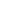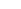# Kleene Star Closure of Regular Grammars

## Algorithm

Given regular grammar G, we would like to construct a regular grammar G' such that L(G') = (L(G))*:

1. construct the kleene plus of the regular grammar (see the relevant section);
2. addto the language (see the relevant section)

## Example

Give a regular grammar recognising the Kleene star closure of the language recognised by the following grammar:

 SaA | bB AaA | a Bc | bS

where S is the start symbol.

1. Construct the Kleene plus closure of the language:

 SaA | bB Aa | aA | aS Bc | cS | bS

where S is the start symbol.

2. Addto the language:

 S1| aA | bB SaA | bB Aa | aA | aS Bc | cS | bS

where S1 is the start symbol.

## Exercises

Construct regular grammars which recognise the Kleene star closure of the languages described using the following regular grammars:

1. G = < { a, b, c },
{ S, A, B, C },
 { SaA | bC, AaA | bB |, BbB | b |, CcB | cC |},
S >
2. G = < { a, b, c },
{ S, A, B, C },
 { SaA | bC |, AaA | aS, Bb, CcB | cS },
S >
3. G = < { a, b, c },
{ S, A, B, C },
 { SaA | bC |, AaA | aS, Bb, CcB | cS },
S >
4. G = < { a, b },
{ S, A },
 { SaA | bS, A},
S >
5.  G = < { a }, { S }, { S}, S >